Next: How many facets does Up: Convex Polyhedron Previous: What computer models are   Contents

## How do we measure the complexity of a convex hull algorithm?

To answer this question, we assume the unit cost RAM model, where the computational time is essentially the number of elementary arithmetic operations and the storage for any integer number takes a unit space. See Section 2.14.

There are two approaches to evaluate the complexity of a given convex hull algorithm.

Let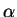be an algorithm which computes a minimal inequality description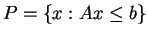of a full-dimensional convex polytopefor a given point set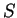in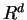with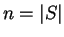. Let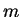denote the number of inequalities in the output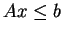.

(One can interprete the discussion here in dual setting: consideras an algorithm to compute all vertices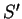of a convex polytopewith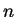inequaities withvertices.)

First of all, most people agree that the efficiency of computing the convex hull should be measured at least by the critical input parameters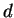and. Some people like to see the complexity by fixingto constant, but it is always better to evaluate in terms ofas well, and fix it later.

The first measure, often employed by computational geometers, is to bound the worst case running time of an algorithmfor any input withpoints in. For example, ifis of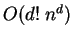, then it meansterminates in timefor ANY input ofpoints in dimension. Also, when one setto be fixed (constant), such an algorithm is said to have time complexity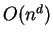, since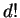is simply a constant. We may call this worst-case-input measure. For fixed dimension, there is an optimum algorithm [Cha93] for the convex hull in terms of the worst-case-input measure, that runs in time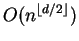for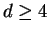. It cannot be better because the largest output is of the same order by the upper bound theorem (Theorem 2).

The worst-case-input measure is quite popular, but it might be little misleading. For example, suppose algorithmsand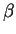are of time complexityand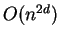, respectively. Then by this measurement, the algorithmis superior to.

Here is a potentially serious problem with this worst-case-input measure. Above, it is still possible thattakes worst-case time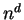for ALL input ofpoints in, andtakes time proportional to some polynomial function of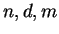. Note that the numberof inequalities varies wildly from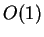to, even for fixed(by the upper bound theorem Theorem 2 and (1)). This diversity is just too big to be ignored if. Furthermore, the input data leading to the worst-case output hardly occurs in practice. In fact, for the random spherical polytope, the expected size ofis linear in, see Section 2.16. While the worst-case-input optimal algorithm [Cha93] is a remarkable theoretical achievement, we are still very far from knowing the best ways to compute the convex hull for general dimensions.

In order to circumvent this pitfall, one can use a measure using all key variables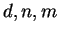. Or more generally, one can measure the time complexity in terms of both the size of input and the size of output. We say an algorithmis polynomial if it runs in time bounded by a polynomial in. This polynomiality coincides with the usual polynomiality when the output size is polynomially bounded by the size of input.

Under the nondegeneracy assumption (see 2.12), there is a polynomial algorithm for the convex hull problem. Few of the earlier polynomial algorithms are pivot-based algorithms [CCH53,Dye83] solving the problem in dual form (the vertex enumeration problem) and a wrapping algorithm [CK70]. A more recent algorithm [AF92] based on reverse search technique [AF96] is not only polynomial but compact at the same time. Here, we say an algorithm is compact if its space complexity is polynomial in the input size only.

In the general case, there is no known polynomial algorithm. The paper [ABS97] is an excellet article presenting how various algorithms fail to be polynomial, through ingenious constructions of nasty'' polytopes.Next: How many facets does Up: Convex Polyhedron Previous: What computer models are   Contents
Komei Fukuda 2004-08-26